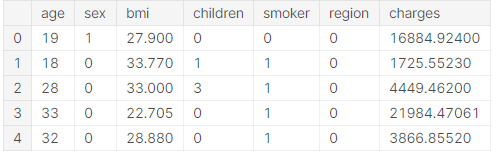# Solving Regression Problems

`Dataset Used: https://www.kaggle.com/mirichoi0218/insuranceMy Notebook: https://www.kaggle.com/tanishsawant2002/regression-85-score`

Description Of The Dataset: (An excerpt from Kaggle)

# Context

Machine Learning with R by Brett Lantz is a book that provides an introduction to machine learning using R. As far as I can tell, Packt Publishing does not make its datasets available online unless you buy the book and create a user account which can be a problem if you are checking the book out from the library or borrowing the book from a friend. All of these datasets are in the public domain but simply needed some cleaning up and recoding to match the format in the book.

# Content

Columns

• age: age of primary beneficiary
• sex: insurance contractor gender, female, male
• bmi: Body mass index, providing an understanding of body, weights that are relatively high or low relative to height,
objective index of body weight (kg / m ^ 2) using the ratio of height to weight, ideally 18.5 to 24.9
• children: Number of children covered by health insurance / Number of dependents
• smoker: Smoking
• region: the beneficiary’s residential area in the US, northeast, southeast, southwest, northwest.
• charges: Individual medical costs billed by health insurance

# Acknowledgements

The dataset is available on GitHub here.

# Inspiration

Can you accurately predict insurance costs?

Ok, Enough about the dataset, now let’s get going with Pandas.

Let’s import the data as a pandas DataFrame

`df = pd.read_csv("/kaggle/input/insurance/insurance.csv")df.head(10)`

I usually prefer to store the column names as a list. (As some features might be changed in future).

`cols = list(df.columns)`

Describe the numerical features

`df.describe().T`

Checking for missing values

`df.isna().sum()`

There are no missing values in the dataset🥳🥳

We can have a quick look at the relation between each features by using seaborn’s pairplot function.

(Seaborn is a Python data visualization library based on matplotlib. It provides a high-level interface for drawing attractive and informative statistical graphics.)

`import seaborn as snsimport matplotlib.pyplot as pltsns.pairplot(df)plt.show()`

By observing the dataset, it is clear that the “sex”, “region” and “smoker” are string datatype columns and can be one hot encoded.

Panda’s get_dummies() method can be used for this purpose.

`df["sex"] = pd.get_dummies(df["sex"])df["smoker"] = pd.get_dummies(df["smoker"])df["region"] = pd.get_dummies(df["region"])df.head()`Now the data is nicely encoded.

We are done with preprocessing and ready to go for building the model.

But wait, one more crucial step is remaining. (Split the dataset)

All columns except “charges” can be added into features table.

`X = df[set(cols)-set(["charges"])]y = df["charges"]`

Splitting data into train and test:

`from sklearn.model_selection import train_test_splitX_train, X_test, y_train, y_test = train_test_split(X, y, test_size=0.33)`

Building Models:

Here I have appended many models to the array so that it get easy to compare the performance. Generally, regression score is measured in terms of r2_score or squared error.

`from sklearn import linear_modelreg = linear_model.LinearRegression()elas = linear_model.ElasticNet()lasso = linear_model.Lasso()huber = linear_model.HuberRegressor()models = [reg, elas, lasso, huber]print(reg)for model in models:    print(model)    model.fit(X_train, y_train)    print(model.score(X_test, y_test))`

Using RandomForestRegressor:

`from sklearn.ensemble import RandomForestRegressorrr = RandomForestRegressor(n_estimators=200, n_jobs = 6, verbose = True)rr.fit(X_train, y_train)rr.score(X_test, y_test)`

Using XGBRegressor:

`xgb = XGBRegressor()xgb.fit(X_train, y_train)xgb.score(X_test, y_test)`

XBGRFRegressor:

`from xgboost import XGBRFRegressorxgbrf = XGBRFRegressor()xgbrf.fit(X_train, y_train)xgbrf.score(X_test, y_test)`

Checking r2_score:

R² (coefficient of determination) regression score function.

Best possible score is 1.0 and it can be negative (because the model can be arbitrarily worse). A constant model that always predicts the expected value of y, disregarding the input features, would get a R² score of 0.0.

`from sklearn.metrics import r2_scorefor model in models:    print("-----------------------------------")    print(r2_score(y_test, model.predict(X_test)))`

Conclusion:

XBGRFRegressor gave the best r2_score.

This was all we need to do😃.

Congratulations! We have successfully built a model to predict the medical charges. 🎉🎊

--

--## TANISH SAWANT

An avid learner who loves exploring the endless world of data science and artificial intelligence. Fascinated by the limitless applications of ML and AI.